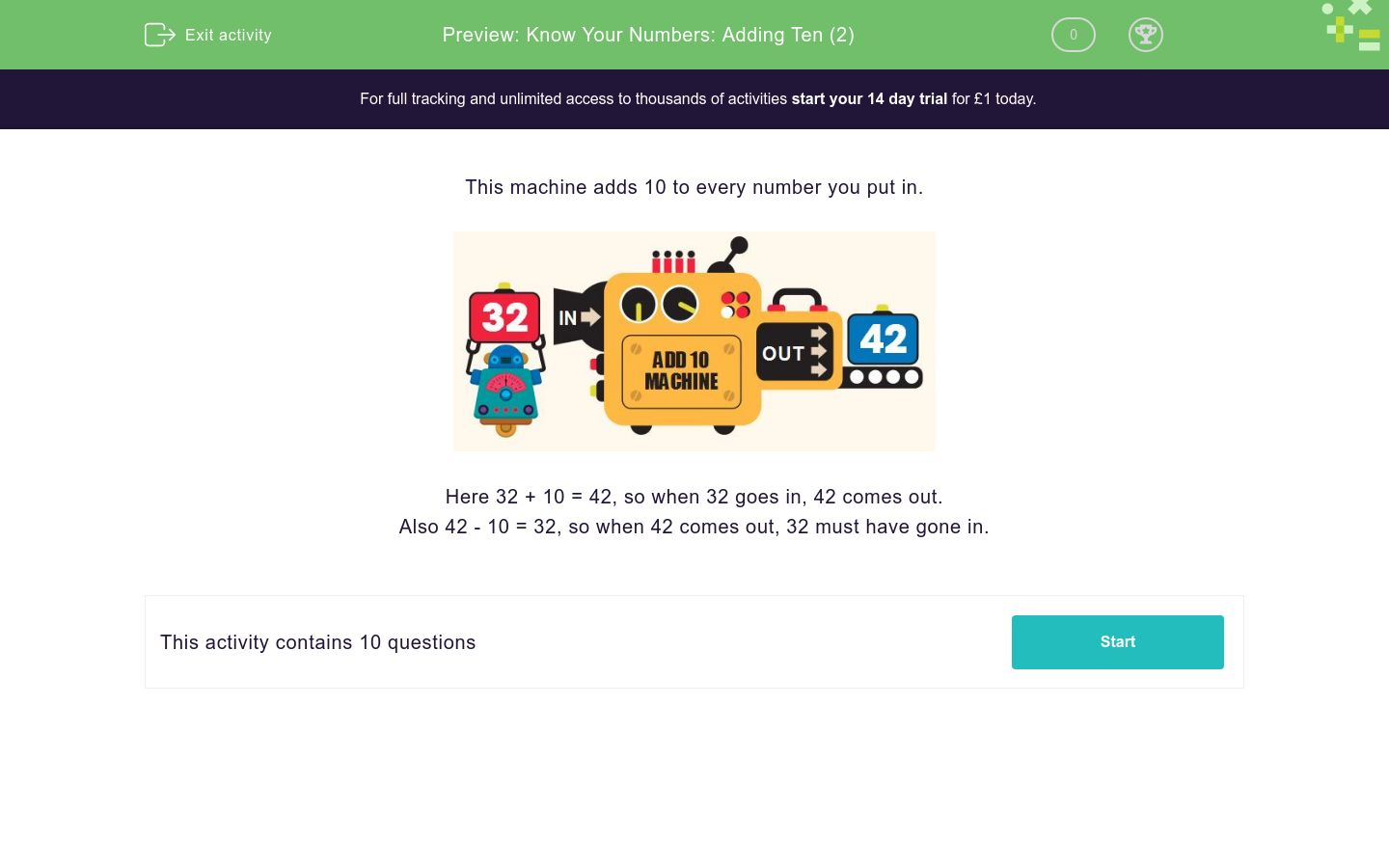### Comprehensive & curriculum aligned

In this worksheet, students add or subtract ten to work out what number goes in to or comes out of the Add Ten Machine.Key stage:

Curriculum topic:

Curriculum subtopic:

Difficulty level:### QUESTION 1 of 10

This machine adds 10 to every number you put in.Here 32 + 10 = 42, so when 32 goes in, 42 comes out.

Also 42 - 10 = 32, so when 42 comes out, 32 must have gone in.14 goes into this machine. What number comes out?16 goes into this machine. What number comes out?38 goes into this machine. What number comes out?29 goes into this machine. What number comes out?47 goes into this machine. What number comes out?22 comes out of this machine. What number went in?45 comes out of this machine. What number went in?29 comes out of this machine. What number went in?67 comes out of this machine. What number went in?99 comes out of this machine. What number went in?• Question 1

14 goes into this machine. What number comes out?24
EDDIE SAYS
14 + 10 = 24
• Question 2

16 goes into this machine. What number comes out?26
EDDIE SAYS
16 + 10 = 26
• Question 3

38 goes into this machine. What number comes out?48
EDDIE SAYS
38 + 10 = 48
• Question 4

29 goes into this machine. What number comes out?39
EDDIE SAYS
29 + 10 = 39
• Question 5

47 goes into this machine. What number comes out?57
EDDIE SAYS
47 + 10 = 57
• Question 6

22 comes out of this machine. What number went in?12
EDDIE SAYS
12 + 10 = 22
or
22 − 10 = 12
• Question 7

45 comes out of this machine. What number went in?35
EDDIE SAYS
35 + 10 = 45
or
45 − 10 = 35
• Question 8

29 comes out of this machine. What number went in?19
EDDIE SAYS
19 + 10 = 29
or
29 − 10 = 19
• Question 9

67 comes out of this machine. What number went in?57
EDDIE SAYS
57 + 10 = 67
or
67 − 10 = 57
• Question 10

99 comes out of this machine. What number went in?89
EDDIE SAYS
89 + 10 = 99
or
99 − 10 = 89
---- OR ----